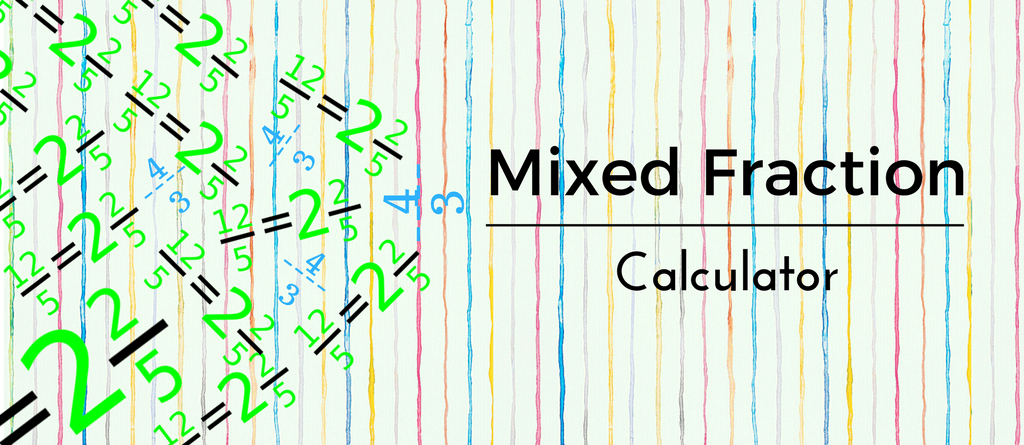Mixed up about mixed fractions? Worry not. This online mixed fraction calculator is here to help. Just put the numbers in the spaces below for adding or subtracting mixed fractions. Yes! It is that simple. So, why wait? Let’s get started.

## Mixed Fraction Calculator

Let’s take an example:

 2 1 + 3 1 4 2

Solution:
To solve a mixed fraction we first have to convert it to an improper fraction

#### Step 0:

Multiply the denominator with the whole number and add the numerator to the multiplication

 (2 x 4) + 1 = 9 4 4
 (3 x 2) + 1 = 7 2 2

#### Step 1:

Equate the denominators
4 x 2 = 8

#### Step 2

(9 x 2) + (4 x 7) = 18 + 28 = 46
Resulting fraction –

 46 8

Simplify

 23 4

### Subtraction

Let’s take an example:

 3 1 – 2 1 2 4

Solution:
To solve a mixed fraction we first have to convert it to an improper fraction

#### Step 0:

Multiply the denominator with the whole number and add the numerator to the multiplication

 (3 x 2) + 1 = 6 2 2
 (2 x 4) + 1 = 9 4 4

#### Step 1:

Equate the denominators
4 x 2 = 8

#### Step 2

Cross-multiply the fraction and subtract
(6 x 9) – (2 x 4) = 54 – 8 = 46
Resulting fraction –

 46 8

#### Step 3:

Simplify

 23 4

Hope this calculator helped. But why just stop here? There are more FREE online calculator for you. Check them out:

Hope this Mixed Fraction Calculator helped. Don’t forget to share with your friends.